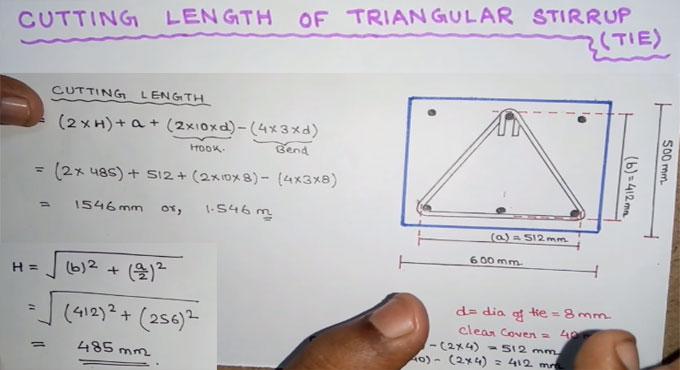# Cutting Length of Triangular StirrupsIt is an easy drawn way with formulas about calculating the cutting length of the Triangular Stirrups. Follow the link for the video.

To calculate the cutting length of Triangular Stirrups first a column need to be drawn. Then with a diagram of 8mm a triangle is drawn into the column. The length of the column is 600mm and the height is 500mm.

The main bar with the black dotted need to be found out. The center to centre of the triangle is dotted and the height and weight need to calculate. By applying the formulas and the drawn figure you can calculate it correctly.